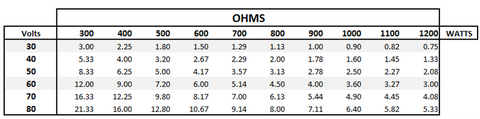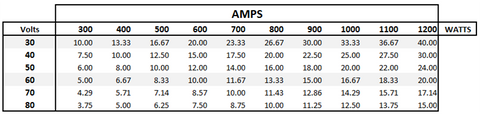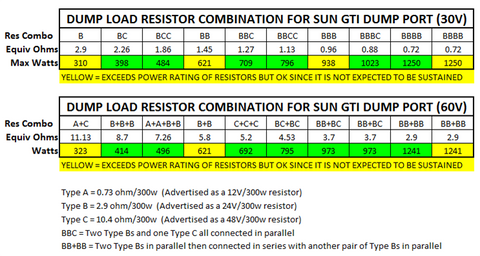# Wind Turbine Grid Tied Divert Load Sizing

He we discuss how to choose a proper divert (dump) load set-up for a wind turbine grid tie system. Different grid ties divert/dump at different voltages and the dump loads must be matched to this voltage. Here we break down simple and easy ways to design the right dump load system for your rig!

The purpose of a divert load in a grid tied system is twofold. First, it provides a method to prevent a turbine from having its RPM run away unrestrained if the grid ever goes down. Secondly, all grid tie systems have a maximum DC input voltage they can tolerate, above which a load needs to be activated to prevent the turbine’s RPM from overspeeding.

It can be a bit complicated to determine what components to buy for a given combination of divert voltage, turbine amps, and divert power. If done incorrectly the divert function may not perform as required; it can overheat and fail and/or it can actually damage the turbine.

These three aspects need to be known:

### Divert Voltage

This is the maximum Grid Tie Inverter (GTI) operating voltage. For instance a Sun-G 22-60V GTI would activate its divert relay at 60 volts.

### Turbine Amps

This at the maximum sustained amps that the turbine can operate at continuous duty. This value can be obtained from the supplier. For the Windy Nation WindTura 750 PMA for instance, this is 40 amps.

### Divert Power

This is the maximum sustained watts that the turbine can operate at continuously. This value can be obtained from the supplier. For the WindTura 750 PMA, this is 1100 watts.

With these values, we can calculate the load resistance in Ohms that is needed as well as the wattage level required for the components.

For example, if a GTI has a 60 volt divert voltage and the Divert Power is 1100 watts, then using Ohm’s law we can solve for Ohms.

P = V2/R, therefore R=V2/P, so R= 602/1100 = 3.27 ohms.

Please note that the letters above represent the following:

• P is Power in Watts
• V is Voltage in Volts
• R is Resistance in Ohms
• I is Current in Amps

We also can check to see if this size resistor will cause more amps to flow than what the PMA can tolerate. Again, using Ohm’s law; I = V/R therefore I = 60/3.27 = 18.33 amps. Since a WindTura 750 PMA can tolerate 40 amps, this 18.33 amp level is very safe to use.

The current value in amps that we just calculated (18.33 Amps) also indicates what the divert relay, circuit, and wiring will have to safely operate at. Check to make sure your divert system can operate well above this level of current for extended periods. Use the National Electrical Code to select the correct wire size.

The following tables illustrate the divert resistor size in ohms and the related amps for various Divert Voltage and Divert Power levels. See if you can find the value for ohms and amps discussed in the above example.

### Divert Resistance

(Example: Suppose your GTI diverts at 60 volts and the maximum sustained power your wind turbine can produce is 1100 Watts. Then you can use the chart below to see that you need a 3.27 Ohm resistor.)### Divert Current

(not to exceed turbine maximum sustained amps)

(Example: Suppose your GTI diverts at 60 volts and the maximum sustained power your wind turbine can produce is 1100 Watts. Then you can see if you use a 3.27 ohm resistor that the divert current (the current traveling through the dump load) is 18.33 amps. You must make sure that this 18.33 amps is well below the maximum sustained current your wind turbine can handle.)Now that we know the resistance and current levels involved, we then need to configure a set of resistors that can provide the target resistance (Ohms) and power dissipation (Watts) levels. Let’s first concentrate on getting the Ohms right. There are 3 situations:

### Resistors in series

In this case, the ohm values are simply added together.

R(total) = R1 + R2 + R3

### Resistors in parallel

This is a bit more complicated, but the basic formula involves adding up the reciprocal of each value and then taking the reciprocal of the sum:

1/ Rtotal = 1/R1 + 1/R2 + 1/R3 +1/R4

For instance a 1 ohm, 2 ohm, and 3 ohm resistor wired in parallel = 0.5454 ohms

### Resistors in series and parallel

This is simply a combination of the above 2 configurations.

It is important to get the total resistance value within 10% of the target value, and it can take a combination of off-the-shelf power resistors to accomplish this.

For instance, WindyNation sells three power resistor values; 0.73 ohms, 2.9 ohms, and 10.4 ohms. Depending on the target ohm value, one or more of these may be required.

If we take the 1100 Watt example of the WindTura 750 PMA operating at 60V and 18.33 amps, we know we need a resistor configuration of 3.27 ohms. Clearly there are multiple mathematical ways to get close using the three resistors mentioned above. For example, two 0.73 ohm resistors wired in parallel which are then wired in series with one 2.9 ohm resistor. The 2 parallel resistors equal 0.365 ohms plus the 2.9 ohms creates 3.265 ohms of resistance. This seems great for the ohms part but we now have a huge new problem -- the power dissipation is inadequate.

Let’s do the math. The two parallel resistors have the capability to dissipate 600 watts, but ohms law indicates they will only dissipate P =I^R therefore P = 18.332 x .365 = 122 Watts, which is 61 Watts each while the single 2.9 ohm resistor will have to then dissipate 18.332 x 2.9 = 974 Watts! Yes, the total is about 1100 watts but the 2.9 ohm resistor will burn up. Yikes!

The point is that we have to solve a small math puzzle to get the resistance to come within 10% of our goal, the total divert power to be met, and the power dissipation of each resistor to be safely within its capabilities.

There are numerous power resistors on the market that can be used. Just follow the steps above.

We sell one type of power resistor that is available in 3 values. These are 300 Watt power resistors. The following resistor combination table has been developed for the 30 volt and the 60 volt divert voltage cases using these resistors. Type A = 0.73 Ohm, Type B = 2.9 Ohm, and Type C = 10.4 Ohm.

(Example: Suppose you are using the 30V GTI and the maximum sustained power your wind turbine can produce is 600 Watts. Then you can use the chart below to see two B resistors (two 2.9 Ohm resistors) wired in parallel will be an adequate divert load for your wind turbine.)